# Fermat-Goss-Denis theorem

(diff) ← Older revision | Latest revision (diff) | Newer revision → (diff)

Fermat's last theorem is the claim that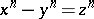has no solutions in non-zero integers for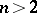(see also Fermat last theorem). However, over a function field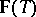(cf. also Algebraic function), with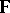of non-zero characteristic, the appropriate generalization is not just to take,andas polynomials overin. In any event, in characteristic zero, or forprime to the characteristic, it is fairly easy to see, by descent on the degrees of a putative solution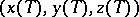, that there is not even a non-trivial solution over, with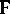the algebraic closure of.

In 1982, D. Goss [a1] formulated a suitable analogue for the case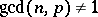. Goss notes that, traditionally, Fermat's equation is viewed as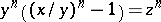, where the connection with cyclotomic fields, and thence the classical exponential function, is displayed: the zeros of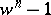are precisely the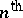roots of unity. But in characteristic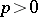the analogue of the exponential function comes by way of the Drinfel'd module; more specifically, the Carlitz module. A familiar and elementary manifestation of such things is the Hilbert theorem 90, whereby a cyclic extension of degreein characteristicis not given by a zero of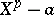, but of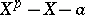.

Let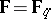be the field of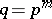elements. The equation that in this context appears to raise issues analogous to those provoked by the classical Fermat equation is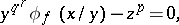whereis the Carlitz module determined by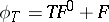anddenotes the Frobenius mapping relative to, i.e. the mapping that gives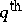powers. To say thatis the Carlitz module is to require also that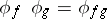. Goss [a1] deals, à la Kummer, with the case of this equation whenis a regular prime of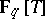.

The equation has two important parameters, the elementofand the order. As usual, a solution with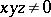is called non-trivial. When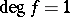, Goss shows that in analogy with the equation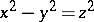there are an infinity of solutions. Supposeis monic. L. Denis [a2] proves that if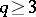,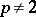and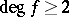, there is no non-trivial solution. If,and, there is a unique solution proportional into the triplet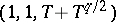in the case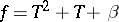, whereis a square in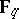; and if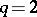,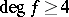, then there is a solution only ifis of the shape, and it is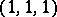. Denis deals completely with the remaining cases. Becauseis-linear, one can now easily produce the results fornot monic.

In settling the general case, Denis [a2] speaks of the Fermat–Goss theorem. It seems appropriate here to write of the Fermat–Goss–Denis theorem.

How to Cite This Entry:
Fermat-Goss-Denis theorem. Encyclopedia of Mathematics. URL: http://encyclopediaofmath.org/index.php?title=Fermat-Goss-Denis_theorem&oldid=16667
This article was adapted from an original article by Alfred J. van der Poorten (originator), which appeared in Encyclopedia of Mathematics - ISBN 1402006098. See original article